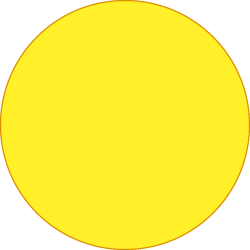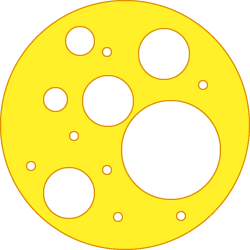# The Serendipity of Swiss Cheese – Evelyn Lamb May 3, 2019

## This holey mathematical object was discovered by a Swiss mathematician and then forgotten for decades

Approximation is a recurring theme in mathematics. Sometimes it seems like all of mathematics is saying, “Well, I know how to solve the problem in this domain. Is there a way I can approximate other domains with this domain?” A lot of calculus boils down to approximating arbitrary functions with linear functions. (For our purposes, “linear” means “completely well-behaved.”) Fourier analysis, which forms the backbone of signal processing, is all about approximating complicated periodic functions with sums of simple sine waves. Scores of mathematicians spend their time thinking about what conditions allow us to approximate functions by simpler functions. A mathematical space called Swiss cheese is yet another example of the ubiquity of approximation.

Like the deli counter staple, mathematical Swiss cheeses are full of holes. To make a Swiss cheese, we start with a closed disc or filled-in circle, the set of all points in the plane that are less than or equal to a given distance (the radius) from a central point.What a lovely, cheesy disc! Credit: Evelyn Lamb

Now we punch it full of holes in a particular way. We want to remove open discs (filled-in discs, but without their boundaries), and we have some rules about the removed discs. If you look up definitions of Swiss cheeses in math, you will see a various definitions, but just like Emmentaler and Gruyére, they are just different particular varieties that all count as Swiss cheese.

This being theoretical math, we remove an infinite number of discs, but we require that the sum of the areas of the removed discs is less than the area of the original disc (often quite a bit less), that no two removed discs overlap, and that the resulting space has no interior. That is, if we zoom in on any part of the space, no matter how small, there are holes in it. It’s hard to imagine such a space (and impossible to draw it, which didn’t stop me), but there are ways to make sure the definition works mathematically.An approximation of a mathematical Swiss cheese. It’s very difficult to draw a picture with interesting features at arbitrarily small scales. Credit: Evelyn Lamb

Swiss mathematician Alice Roth, the first person to describe these spaces, was trying to understand the limits of approximations of continuous functions by rational functions in the complex plane. A rational function is the ratio of two polynomials. Polynomials might be familiar to you from algebra classes. They are some of the simplest and easiest to understand functions. They have expressions like z+5 or z3-9z+7. Rational functions have expressions like (z3-9z+7)/(z+5).

Is it always possible to approximate continuous functions by rational functions, or are there some spaces where this fails? Roth and other mathematicians showed that there are continuous functions on Swiss cheeses that cannot be approximated by rational functions.

I am both a mathematician and a lover of cheese, so when I first heard that there were mathematical objects called Swiss cheeses, I was intrigued. The related research paper titles were delightful. Abstract Swiss Cheese Space and the Classicalisation of Swiss Cheeses. Swiss cheeses, rational approximation and universal plane curves. On the volume of the intersection of two Wiener sausages. (That last paper describes Swiss cheese-related calculations on another punny mathematical space called a Wiener sausage. Mathematicians like food.) Everything about this was relevant to my interests. When I learned about the history of the name, it got even better.

Article continues: